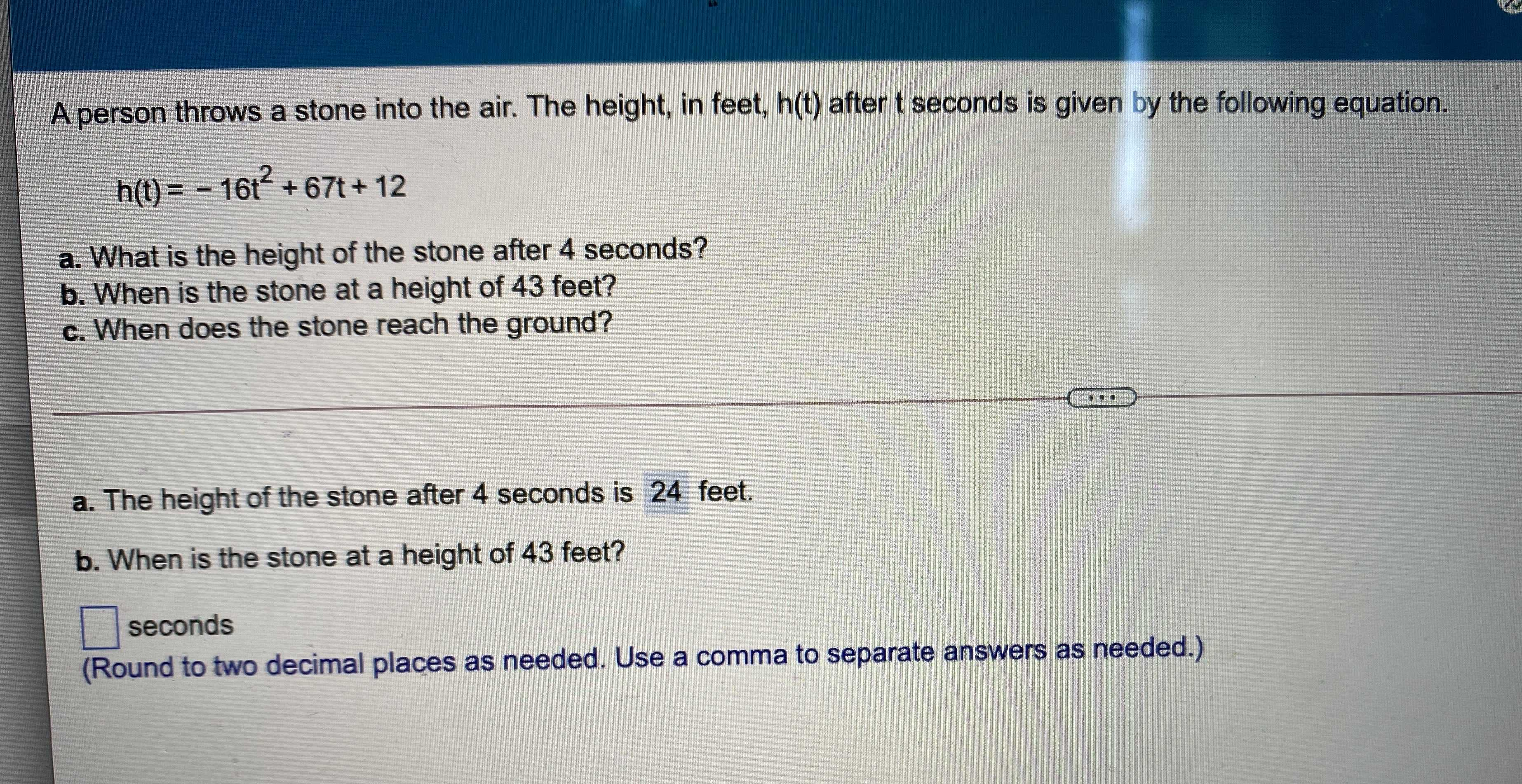### ¿Todavía tienes preguntas de matemáticas?

Pregunte a nuestros tutores expertos
Algebra
PreguntaA person throws a stone into the air. The height, in feet, $$h ( t )$$ after $$t$$ seconds is given by the following equation.

$$h ( t ) = - 16 t ^ { 2 } + 67 t + 12$$

a. What is the height of the stone after $$4$$ seconds?

b. When is the stone at a height of $$43$$ feet?

c. When does the stone reach the ground?

a. The height of the stone after $$4$$ seconds is $$24$$ feet.

b. When is the stone at a height of $$43$$ feet?

____seconds

(Round to two decimal places as needed. Use a comma to separate answers as needed.)